# Knot at Lunch, June 28, 2007

## Invitation

Dear Knot at Lunch People,

We will have the fourth of our weekly summer lunches on Thursday June 28, 2007, at the usual place, Bahen 6180, at 12 noon, though this time it will be a short meeting - I'd like to attend a lecture at the Fields Institute at 1PM (titled "Proof of Banach-Tarski paradox") so we have to finish before that.

As always, please bring brown-bag lunch and fresh ideas. I have a few ideas for the agenda: a bit of propaganda about computing, and some words about an email I sent Dylan a few days ago (attached below). But of course, we may end up talking about something else.

As always, if you know anyone I should add to this mailing list or if you wish to be removed from this mailing list please let me know. To prevent junk accumulation in mailboxes, I will actively remove inactive people unless they request otherwise.

Best,

Dror.

Dylan,

In all things finite type (and elsewhere in knot theory) braids have always been one of the simplest examples.

So here's a bunch of *specific* questions:

What are virtual braids? Can they be combed? What are finite type invariants of virtual braids? Is there a structure-preserving universal finite type invariant of virtual braids? Does it separate virtual braids? Does every finite type invariant of braids extends to a finite type invariant of virtual braids, of the same degree? Do "Lie-bialgebraic" weight systems span all weight systems, for virtual braids? Can you write honest formulas in terms of arrow diagrams for an R-matrix?

Dror.

## Some Content

 When will it finally be easy to draw on a computer?

Definition. The pure virtual braid group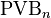$\operatorname{PVB}_n$ on$n$ strands is the group generated by symbols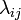$\lambda_{ij}$ for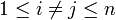$1\leq i\neq j\leq n$ (standing for "strand$i$ goes over strand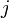$j$ from λeft to right and returning virtually") and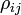$\rho_{ij}$ (standing for "strand$i$ goes over strand$j$ from ρight to left, virtual return") modulo the relations:

•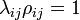$\lambda_{ij}\rho_{ij}=1$.
•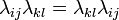$\lambda_{ij}\lambda_{kl}=\lambda_{kl}\lambda_{ij}$ (where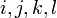$i,j,k,l$ are distinct; variants: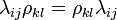$\lambda_{ij}\rho_{kl}=\rho_{kl}\lambda_{ij}$ and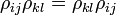$\rho_{ij}\rho_{kl}=\rho_{kl}\rho_{ij}$).
•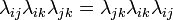$\lambda_{ij}\lambda_{ik}\lambda_{jk}=\lambda_{jk}\lambda_{ik}\lambda_{ij}$ (where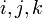$i,j,k$ are distinct; variants?).
 When will it finally be easy to draw on a computer?

At least at a naive level, the corresponding algebra of chord diagrams is generated by degree 1 symbols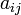$a_{ij}$ (corresponding to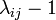$\lambda_{ij}-1$) and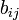$b_{ij}$ (corresponding to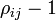$\rho_{ij}-1$) subject to the relations:

•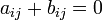$a_{ij}+b_{ij}=0$ (and hence the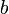$b$'s can henceforth be ignored).
•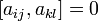$[a_{ij},a_{kl}]=0$.
•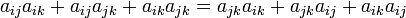$a_{ij}a_{ik}+a_{ij}a_{jk}+a_{ik}a_{jk} = a_{jk}a_{ik}+a_{jk}a_{ij}+a_{ik}a_{ij}$.